﻿ 随机森林储层预测及关键参数探讨——以SC某工区储层预测为例

# 随机森林储层预测及关键参数探讨——以SC某工区储层预测为例Random Forest Reservoir Prediction and Key Parameters Discussion—Taking Reservoir Prediction of a Work Area in SC as an Example

Abstract: Random forest is a highly flexible machine learning algorithm, which can solve the problems of regression and classification. According to the process of random forest seismic reservoir prediction, this paper discusses the key parameters in the process of random forest reservoir prediction. Taking the reservoir gamma prediction of a work area in SC as an example, the influence of different parameters on the prediction results is compared and analyzed, and the optimal parameter settings are given. At the same time, the importance of seismic attributes is analyzed, and the optimal attribute sample set is obtained. The optimal random forest model is used to carry out reservoir prediction with the optimal attribute as the input, and good results are achieved. It is proved that random forest algorithm can effectively predict reservoir. Parameter optimization of random forest model is very important, which affects the efficiency and final prediction accuracy of the algorithm.

1. 引言

2. 方法原理

2.1. 随机森林算法

2.1.1. 随机森林原理及特征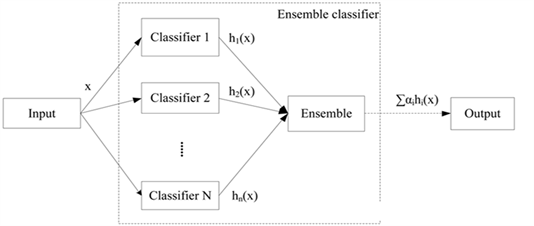Figure 1. Schematic diagram of ensemble learning

2.1.2. 随机森林模型构建

1) 构建初始模型

2) 特征集选择

$\text{M}\left({Y}_{i}\right)=\frac{1}{{N}_{i}}{\sum }_{t=1}^{{N}_{i}}\left(errOO{B}_{t1}?errOO{B}_{t2}\right)$ (1)

3) 优化随机森林

2.2. 随机森林储层预测

1) 层位标定。

2) 数据重采样。

3) 样本集确定。

4) 随机森林模型建立。

5) 储层参数预测。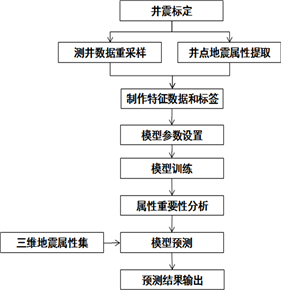Figure 2. Flow chart of reservoir prediction using Random Forest

3. 应用实例Table 1. Training sample data set (The first seven lines)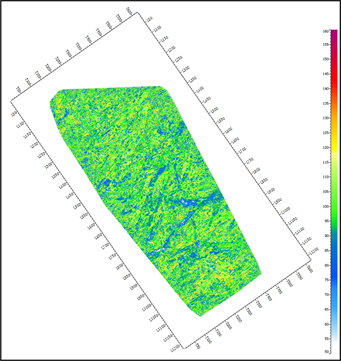(a) 树个数 = 5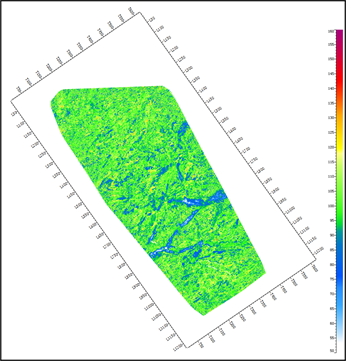(b) 树个数 = 20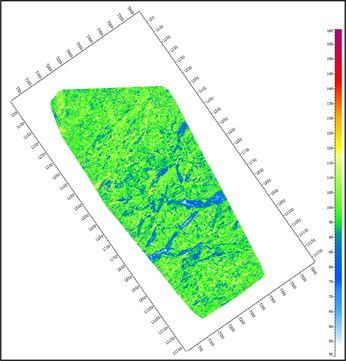(c) 树个数 = 50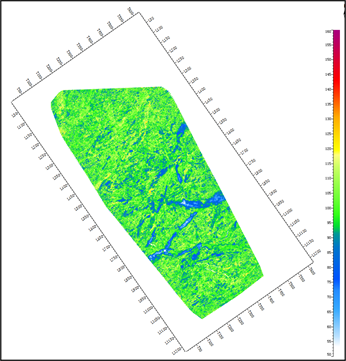(d) 树个数 = 80

Figure 3. Comparison of different numbers of decision trees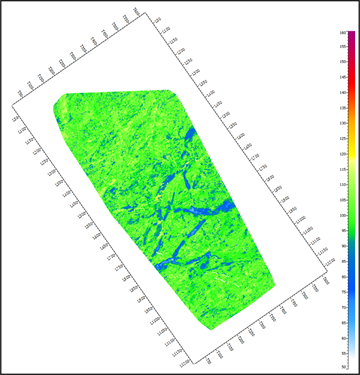(a) 最大深度 = 5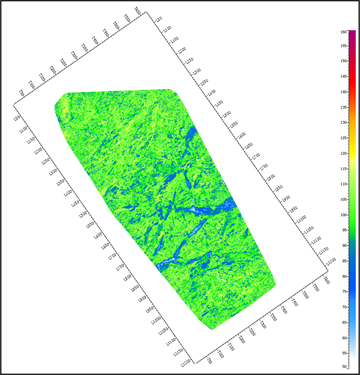(b) 最大深度 = 10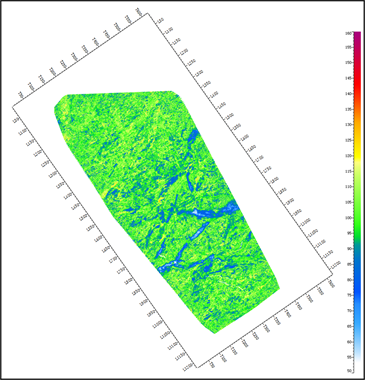(c) 最大深度 = 20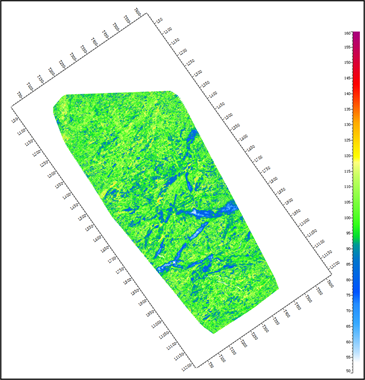(d) 最大深度 = 不限制

Figure 4. Comparison of different maximum depth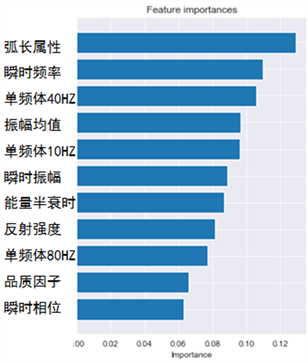Figure 5. Comparison chart of importance of attributesFigure 6. Comparison of results before (left) and after (right) attribute optimization

4. 结论

1) 随机森林可以解决回归问题(储层物性参数预测)和分类问题(地震相划分、岩性识别)，实践证明随机森林模型预测结果良好。

2) 随机森林算法可以对特征进行重要性分析，即对参数计算的不同类型的地震属性进行敏感性分析。敏感性高的属性组合与测井参数具有更准确的非线性映射关系。

3) 随机森林模型的参数调优很重要，影响到算法的效率和预测结果精度，是建模过程中的必须步骤。

 Breiman, I. (2001) Random Forests. Machine Learning, 45, 5-32.
https://doi.org/10.1023/A:1010933404324

 方匡南, 吴见彬, 朱建平, 等. 随机森林方法研究综述[J]. 统计与信息论坛, 2011, 26(3): 32-38.

 Reif, D.M., Motsiniger, A.A., McKinne, B.A., et al. (2006) Feature Selection Using a Random Forests Classifier for the Integrated Analysis of Multiple Data Types. CIBCB, 1-8.
https://doi.org/10.1109/CIBCB.2006.330987

 Diaz-Uriarte, R. and Andres, S.A.D. (2005) Variable Selec-tion from Random Forests: Application to Gene Expression Data. Spanish Bioinformatics Conference.

 张华伟, 王明文, 甘丽新. 基于随机森林的文本分类模型研究[J]. 山东大学学报(理学版), 2006, 41(3): 5-9.

 方匡南. 随机森林组合预测理论及其在金融中的应用[M]. 厦门: 厦门大学出版社, 2012.

 王志宏, 韩璐, 戚磊. 随机森立分类方法在储层岩性识别中的应用[J]. 辽宁工程技术大学学报(自然科学版), 2015, 34(9): 1083-1088.

 宋建国, 高强山, 李哲. 随机森林回归在地震储层预测中的应用[J]. 石油地球物理勘探, 2016, 51(6): 1202-1211.

 柴明锐, 程丹, 张昌民, 朱锐, 唐勇, 瞿建华. 机器学习方法对砂砾岩岩屑成分的预测——以西北缘X723井百口泉组为例[J]. 西安石油大学学报(自然科学版), 2017, 32(5): 22-28.

 周雪晴, 张占松, 张超谟, 聂昕, 朱林奇, 张宏悦. 基于粗糙集——随机森林算法的复杂岩性识别[J]. 大庆石油地质与开发, 2017, 36(6): 127-132.

 高强山. 基于随机回归森林的储层预测方法研究[D]: [硕士学位论文]. 青岛: 中国石油大学(华东), 2017.

 何健. 基于随机森林算法的储层预测[D]: [硕士学位论文]. 成都: 成都理工大学, 2020.

 Brown, A.R. (2001) Understanding Seismic Attributes. Geophysics, 66, 47-48.
https://doi.org/10.1190/1.1444919

 Chen, Q. and Sidney, S. (1997) Seismic Attribute Technology for Reservoir Forecasting and Monitoring. The Leading Edge, 16, 445-448.
https://doi.org/10.1190/1.1437657

 Taner, M. (2001) Seismic Attributes. CSEG Recorder, 26, 8-56.

 魏佳明, 韩家新. 随机森林在储层孔隙度预测中的应用[J]. 智能计算机与应用, 2018, 8(5): 79-82.

Top